# Solution assignment 07 Unit circle and simple formulas

### Assignment 7

Give the period of: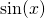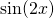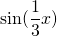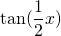### Solution

The period ofequals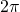(after one turn we start again). In other words: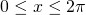For the functionwe have: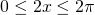or: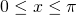and thus the period of this function is equal to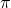.
For similar reasons the other functions have, subsequently, the following periods: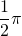en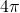0